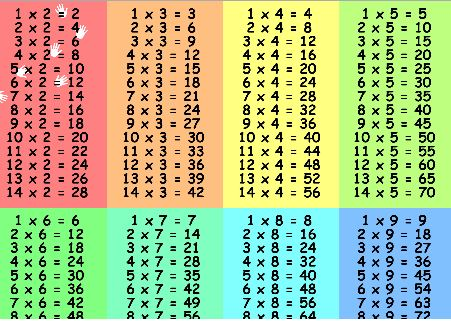# Not your average table of operationsConsider the 10-digit integer

$N=\overline { ABCDEFGHIJ}$

(where $A,B,C,D..J$ are the digits of $N$). Each digit of $N$ is a number between $0$ and $9$ inclusive and all digits of $N$ are different from each other.

What must be the value of $N$ so that the three vertical and three horizontal operations in the table below are correct?

$\begin{matrix} \overline { EDJH } & \div & \overline { JF } & = & \overline { AA } \\ - & \quad & + & \quad & + \\ \overline { EDB } & \times & \overline { I } & = & \overline { EHCG } \\ \quad = & \quad & = & \quad & = \\ \overline { EEID } & - & \overline { DJ } & = & \overline { EEAE } \end{matrix}$

Details and assumptions

You may assue that only one value of $N$ satisfies the constraints above.

×

Problem Loading...

Note Loading...

Set Loading...